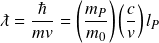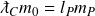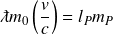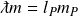# Rest mass operator

The rest mass operator describes the influence of rest mass on wave-like particles. Rest mass is a quantity of latent energy equal to the field resistance imposed on some particles. Rest mass has the following effects that are quantified by the operator:

1. Rest mass reduces a particle’s inertial mass and energy potentials. Whereas particles without rest mass have Planck scale potentials, particles with rest mass are limited to the scale of a particle’s Compton wavelength. Inertial mass, velocity, and kinetic energy are all maximized at this scale.
2. Rest mass partitions a particle’s energy between inertial mass and velocity, generating equal ratios of 𝑚/𝑚0 and 𝑣/𝑐.

The following illustrations compare the potentials of particles with and without rest mass. The impact of rest mass is seen on the right where the particle’s wavelength cannot reach the Planck scale, but reaches its maximum potential at the scale of the particle’s Compton wavelength.

### Rest mass

The rest mass operator is a value between 0 and 1 representing the inverse number of natural length units comprising the particle’s Compton wavelength. Dividing the number 1 by the rest mass operator gives the Compton wavelength in natural length units.

The New Foundation Model shows the rest mass operator as a single line across the three unit dimensions. The potential in each unit dimension is reduced proportionally.

The three correlated unit dimensions give three equivalent forms of the rest mass operator–one in each unit dimension:

## Relationship between wavelength and inertial mass

The relationship between a particle’s wavelength and inertial mass can be understood through the elementary forms of the Compton and de Broglie wavelength formulas

### Compton wavelength### de Broglie wavelengthElementary forms of the Compton and de Broglie wavelength formulas show a dual meaning for the Compton wavelength. For particles without rest mass, including photons, the Compton wavelength gives a quantity of energy equal to the rest mass of a massive particle through inelastic scattering. But the Compton wavelength is also the wavelength of a massive particle at the limit of its velocity potential 𝑐. The two formulas coincide at this limit.

The de Broglie wavelength formula is generally applied to particles with rest mass, but the formula works for all particles when we apply the concept of inertial mass. Any given wavelength for either type of particle produces the same quantity of inertial mass.

We can treat the de Broglie formula as a general formula for all particles with respect to their inertial mass, and the Compton wavelength as a special case of the same formula at the maximum velocity potential (see examples of photon and electron mechanics).

The Compton wavelength also quantifies a particle’s rest energy which is the particle’s maximum energy potential at the speed of light.

The two wavelength formulas show a conserved relationship between wavelength and inertial mass given the constant 𝑙𝑃𝑚𝑃. This relationship is explained by length-mass and mass-momentum symmetries:Replacing rest mass and velocity 𝑚0𝑣 with inertial mass 𝑚 gives the general formula that is true for all particles:We can also show this relationship as an equality between the length and mass forms of the wavelength operator. The Compton wavelength formula on the left is just one combination of wavelength and mass that is captured by the general de Broglie formula on the right.## Rest mass versus inertial mass

Rest mass is an equilibrium between the resistance a particle feels from its interaction with the Higgs field and the energy it possesses to meet that resistance. This equilibrium between opposing forces consumes a substantial portion of the particle’s conserved energy potential, preventing its wavelength from becoming shorter than the Compton wavelength (in non relativistic terms).

Inertial mass is an amount of energy potential inversely proportional to a particle’s wavelength. For particles with rest mass, inertial mass and rest mass are equal at the limit of the particle’s velocity potential, the speed of light. But as the particle’s wavelength increases, inertial mass decreases while rest mass remains constant.

The relationship between rest mass and inertial mass is captured by the energy-momentum relationship. The energy momentum formulacan be re-stated in terms of inertial mass in unit dimension M rather than momentum in unit dimensions LMT-1. The natural formuladescribes the energy-momentum relationship as the sum of two mass vectors contributing to the particle’s total energy.

We can think of rest mass as a well or reservoir that must be filled before water can obtain kinetic properties. Given a limited amount of water, the reservoir will consume some portion of the water’s potential energy before any kinetic energy is created. Once the reservoir is full, the remaining water produces kinetic energy.

Inertial mass determines a particle’s kinetic energy in the shape of its wavelength. A particle’s maximum inertial mass is equal to its rest mass at the limit of its shortest possible wavelength–the Compton wavelength. Particles moving at less than light speed have less inertial mass than rest mass.

The New Foundation Model shows that a broader definition of mass is needed, where the current definition of mass is reserved for rest mass. There are compelling reasons to treat inertial mass as a genuine form of mass, including

1. The concept of effective mass is often cited in the context of “massless” particles. It is generally understood that photons carry a measure of strength comparable to particles with rest mass.
2. Planck’s constant, which is used to calculate the momentum and energy of photons, includes the quantity and unit dimension of Planck mass. Inertial mass is the same proportion of Planck mass as wavelength, momentum, and energy are to their Planck values.
3. Inertial mass produces the correct energy of a photon when inserted into Einstein’s mass-energy equivalence formula, 𝐸 = 𝑚𝑐2.

## Relationship with other mechanical operators

The rest mass operator quantifies a portion of a particle’s wavelength, yielding only a fraction of the wavelength component in the 2-part energy mechanism. The remaining portion of the particle’s wavelength is quantified by the momentum operator. The product of these two operators is equal to the wavelength operator.This relationship suggests that rest mass and velocity are simply instruments for calculating a particle’s wavelength, which gives the genuine physical description of its energy potential. The benefit of this approach is a single explanation for the kinetic energies of particles whether rest mass is present or not.

The following illustration shows the relationship between the mechanical operators.

We can also view the relationship in each of the fundamental unit dimensions. For particles with rest mass, the wavelength operator can be split into two parts. The first part, the rest mass operator, is the distance from the Planck length to the particle’s Compton wavelength. The second part, the momentum operator, is the distance from the Compton wavelength to the de Broglie wavelength.

βλ

βm βp

βλ

βm βp

### Time

βλ

βm βp

The relationship between length and mass is exhibited in the rest masses and Compton wavelengths of the charged leptons. Applying any form of the operator to maximum length and mass each potentials produces proportional quantities of wavelength and rest mass.

The expanded form of the particle mechanics template replaces the wavelength operator with the combined rest mass and momentum operators.

Rest mass operator